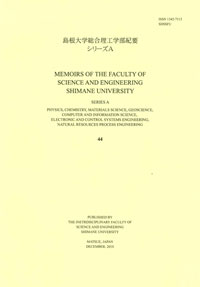# Memoirs of the Graduate School of Science and Engineering Shimane University. Series A

ISSN:13427113Memoirs of the Graduate School of Science and Engineering Shimane University. Series A 33
1999-12-24 発行

# 圧縮-振動複合応カ下におげる木材の力学挙動と圧電現象

Mechanical Behaviror and Piezoelectric Phenomenon of Wood under Combined Compressron and Vibration Stresses
Nakai, Takahisa
Description
This study investigated the relation between the piezoelectric voltage and microscopic fracture of a Hinoki (Chamaecyparis obtusa Endl.), in particular the deformation of the cross-sectional wall of the tracheid under combined compression and vibration stresses, and clarified the relation as described below.
1 . The piezoelectric voltage-displacement (P-D ) curve consisted of a linear region starting from the origin followed by a convex curved region. The linear region of the P-D curve was only about 60 percent of that of the load-displacement (L-D ) curve.
2. By applying stresses to a specimen, the cross-sectional walls of the tracheid were deformed mainly at the radial walls . When a tracheid was regarded approximately as a hexagonal prism, the elastic buckling stress of the cross-sectional wall of the tracheid was estimated from SEM images and our method based on a modificatton of the Gibson and Ashby method. As a result, it was estimated that the elastic buckling stress of a radial wall of tracheid was only about 80 percent of the stress at the proportional limit of the P-D curve .
3. It is found that there are two consecutive regions before proportional limit of the P-D curve: one is the region up to the spot where the radial cell wall generates the elastic buckling, and the other is the region starting from the end of the above region up to the proportional limit of the P-D curve.
4. The P-D curve changed from the linear-elastic region to the convex curved region as soon as a radial wall of the tracheid generated an elastic buckling. A maximal point on the convex curve was observed. The maximal point of the P-D curve coincides with the proportional limit of the L-D curve. After reaching the proportional limit of the L-D curve, the piezoelectric voltage decreased gradually. The L-D curve after the maximal point increased curvedly and shifted toward the plateau part caused by the buckling fracture of the specrmen.
5 . It was supposed that the peak of the P-D curve which appeared in the nonlinear region was the piezoelectric polarization which is caused by the non-uniform local strain.

NCID
AA11157087